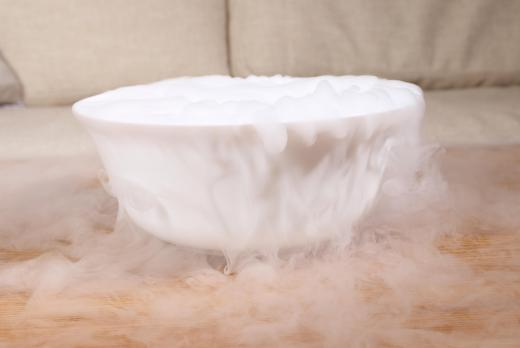# What is a Phase Diagram?

Daniel Liden
Daniel LidenTypically, carbon dioxide goes from a solid phase to a gas phase without a liquid phase in between.

A phase diagram is a chart that is commonly used in the sciences, especially chemistry, to visualize the conditions under which a substances exists in a given phase and changes to another phase. The phases addressed by a phase diagram are typically the solid, liquid, and gaseous phases. The diagrams are set up as graphs with pressure on the vertical axis and temperature on the horizontal axis. Curves on the diagram represent points at which different phases are at a state of equilibrium, and open spaces represent distinct phases. A great deal of valuable information, such as boiling point and freezing point of a substance at a given pressure, can be gathered from such a diagram.

The typical phase diagram will start at very low temperature and pressure and begins with only one curve. Above the curve is the solid phase, and below it is the gaseous phase. The curve itself represents equilibrium between solid and gas; at very low pressure conditions, liquid seldom exists. This equilibrium is also referred to as the sublimation point. Eventually, at a specific temperature and pressure, the curve branches off into two distinct curves, and the space between the two represents the liquid phase.

The point at which the curve branches into two curves is referred to as the triple point. It is important because it represents the specific conditions of temperature and pressure at which the solid, liquid, and gaseous phases are all in a state of equilibrium. This means that the substance represented by the chart is constantly shifting between the phases--all three are present at any given time.

At some temperature and pressure, the lower curve ends at a point referred to as the critical point. This usually occurs at relatively high pressure and temperature. At pressure and temperature conditions higher than the critical point, there is no discernible distinction between the liquid phase and the gaseous phase.

Phase diagrams are useful, and can be very important to chemists. The curve on the phase diagram dividing liquid and gas, for example, represents the conditions under which the two will be at equilibrium. In other words, the curve represents the boiling point of the substance.

## You might also Like